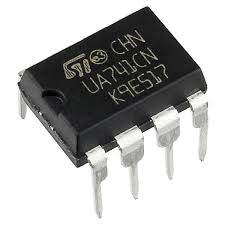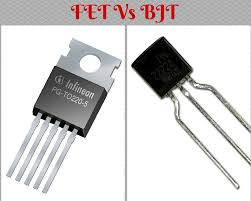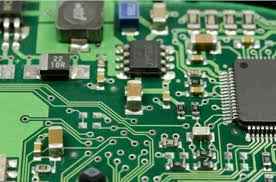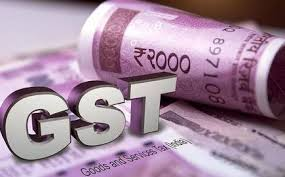Discipline Specific Course

### Chemistry IV

 UNIT OBJECTIVES I Inorganic Chemistry: This course is to study about chemistry coordination complexes, Coordination number and  Isomerism in coordination compounds. II Organic Chemistry:  The course is designed to provide concepts in organic chemistry which includes synthesis and properties of carboxylic acids and nitro compounds. III Physical Chemistry:  This course is designed to provide the basic concepts in physical chemistry which  include Kholrausch’s law, Onsagar’s equation, Nernst equation and its applications. IV General Chemistry:The course is to study classification of  pericyclic reactions, mechanism of the reactions and also include stereochemistry of the reactions(Enantiomers and Diastereomers)

COURSE OUTCOMES:

Students will be able to

CO1: Explain the ligand field splitting of d-orbital in different complexes.

CO2: Discussthe effect of substituents on the acidity of carboxylic acids and phenols.

CO3: Explain the synthetic applications of acetoacetic ester.

CO4: Describe the structure of various metal carbonyl compounds.

CO5: ApplyNernst equation for calculating E.M.F of electrolytic cells.

CO6: Explain the electrocyclic and cycloaddition pericyclic reactions.

CO7: Identify the stereoselective reactions.

### Electronics - VII

 CO1: The students will be able perform the time and frequency domain analysis of the                                  signals in a digital communication system. CO2: The students will be able to a design of digital communication system. CO3: The students will be able to study the and plot the wave structures for different pulse modulations CO4: The students will be able to understand the basics of information theory, source     coding techniques and calculate Entropy of source.CO5: The students will be able to determine the performance of line codes CO6: The students will be able to describe and determine the performance of different error control coding schemes for the reliable transmission of digital signals. CO7: The student will be able to understand various spreading techniques and determine bit error performance of various digital communication systems. CO8 :The students will be able to theoretical concepts of paging system, cellular telephone, global positioning satellite ,facsimile ,video text. CO9: Students will be able to study the properties of Fourier series ,complex Fourier transforms and sampling theorem.### Electronics - IV

CO1:  The students will be able to explain the each block of op amp .

CO2: The students will be able to define a significance of op amp and their importance.

CO3: The students will be able to build circuits using analog ICs.

CO4: The students will be able to study in depth knowledge of applying the concepts in real time applications.

CO5: The students will be able to use Op Amp as summer, subtractor, comparator, integrator ,and differentiator.

CO6: The students will be able to generate a sine wave ,square wave and triangular wave using Op amp.

CO7: The students will be able to explain basic information about communication system and its block diagram and the purpose of modulations and demodulations technique.

CO8: The students will be able to explain about different type of modulators and demodulators, as well as AM and FM transmitters and receivers .### Financial Accounting II### ELECTRONICS DEVICES -Paper II

 CO1:  The students will be able to plot the VI characteristics of all diodes and can design a p-n junction structure given required electrical performance and compare the experimental data to the theoretical curve of the diodes. CO2: The students will be able to use the scanned-load-line methods to obtain the I-V characteristic of the BJTs CO3: The students will be able to design and implement single-stage BJT amplifiers and observe amplitude and frequency response. CO4: The students will be able to calculate the terminal currents : IE, IC, IB CO5: The students will be able to describe the basic FET structure/operation by channel types (n or p), and by the presence/absence of conduction channel  CO6: The students will be able to calculate the threshold voltage (VT) of MOS structures and explain how VT can be controlled CO7: The students will be able to describe the structures and the operating principles of JFETs, MOSFETs and design a MOSFET structure given required electrical performance
CO8: The students will be able to draw practically the characteristics of SCR and UJT and will be able to explain the operating principles, structures, electrical characteristics of various optoelectronic devices like:LEDs, photo detectors , solar cells### ELECTRONICS(Paper - III) :Analog Circuits

COURSE OBJECTIVES:

 UNIT OBJECTIVES I §  Understand the voltage-current characteristics of a semiconductor diode §  Understand operation of half-wave and full-wave rectifier circuits §  Determination of output voltages and currents. §  Analyze the operation of rectifier circuit with capacitor filter §   Calculation of peak inverse voltage for rectifier circuits §  Study the application of diodes in power supply circuits II §  Explain what is meant by the terms load regulation and line regulation; §  Draw the circuit diagram for an emitter follower based on an npn transistor; §  Recall that for an emitter follower: ·          input impedance ~hFE RE ·         VOUT = VIN – 0.7V §  Design and analyse a voltage regulator based on a zener diode, an emitter follower and a non-inverting amplifier §  Select and use the following gain formula to calculate the output voltage:                                                 VL » VZ (1 + RF / R1) III §  Explain how the transistor can be used to amplify a signal. §  Name the four classes of amplifiers and give an explanation for each. §  Explain graphically the amplification process in BJT §  Describe the small signal hybrid equivalent of transistor and determine its parameters §  Define feedback §  Need for feed back §  Types of feedback §  Compare negative and positive feedback. IV §  Describe the basic concept of oscillators §  Analyse the operation of RC and LC oscillators §  Identify the Hartley oscillator. §  Identify the Colpitts oscillator. §  Identify the resistive-capacitive oscillator. §  Determine the frequency of a resistive-capacitive oscillator. §  Describe the differences between series-fed and shunt-fed oscillators. §  Explain how the crystal is equivalent to the series and parallel LC circuit.

COURSE OUTCOMES:

 OUTCOMES CO1:   The students will be able to draw and understand the use of diodes in half wave and full wave bridge rectifiers.   CO2: The students will be able to  calculate the peak value of the output voltage of half wave and full wave rectifiers given the rms input voltage   CO3: The students will be able to  know the principle of operation and working of Transformers and UPS CO4:  The students will be able to test and troubleshoot the Industrial electronic circuits and components CO5: The students will be able to  learn about different types of feedback amplifiers,  Design and analyze the cascaded RC coupled BJT amplifier   CO6: The students will be able to  calculate the voltage gain and to observe frequency response of RC Coupled amplifier   CO7: The students will be able to  analyze and deign op-amp, oscillators ,single chip oscillators   CO8:   The students will be able to demonstrate working of oscillator theory design and build a Colpitts oscillator .### Theory & Practice of GST D### FRENCH SEM I

COMMUNICATION